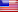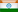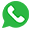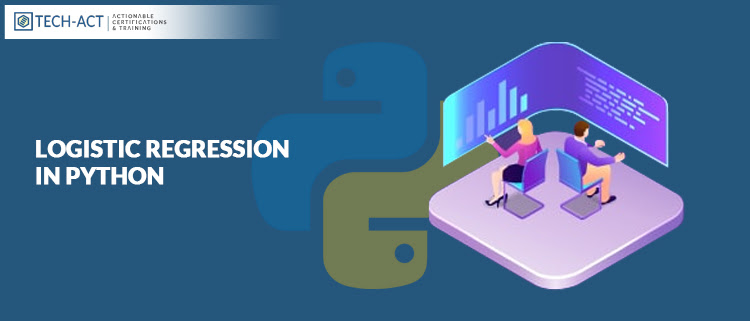(+1) 408-400-3668(+91) 913-696-0293# Logistic Regression In Python

By Tech-Act
06/23/2021  2752 ViewsData plays a major role because if the data is available in huge amount then the strength of computing power & the number of algorithmic improvements keep rising & eventually this increases the importance of data science and machine learning. One of the most important areas in Machine Learning is classification and logistic regression is one of its basic methods.

Today, in this article we will throw light on logistic regression in python packages followed by an illustrative example of logistic regression solved with Scikit-learn. Let’s begin.

#### Logistic Regression Python Packages

• You will need various packages for logistic regression in Python. Well, the good part is that all of these packages have open-source and are free and have ample of resources readily available.
• The first package which you will need is NumPy, as it is the basic package for scientific and numerical computing in Python. A high-performance operation on single- and multi-dimensional arrays is possible by using NumPy and therefore it is also very popular. It offers various useful array routines. Users can write elegant and compact code using NumPy. Also NumPy works very well with Python Packages. NumPy offers exhaustive documentation on its functions, classes & methods.
• Another, python package is scikit-learn, one of the most popular data scienceand machine learning Scikit-learn can be used for carrying out various functions like preprocess data, for reducing the dimensionality of problem, for validating models, for selecting the most appropriate model, for scikit learn logistic regression and classifying problems & for implementing cluster analysis.  You can visit the official scikit-learn website for gaining the information on generalized linear models and logistic regression implementation.
• Some functionalities may lack in scikit-learn so then you will find StatsModels StatsModel is a very sturdy python library for statistical analysis.
• If you want to visualize the output of your classification then you can use Matplotlib. It is very exhaustive and it is used on large scale for high-quality plotting. Different sources are available for learning Matplotlib.

#### Logistic Regression in Python With Scikit-learn

This example is related to a single-variate binary classification problem. It is a very simple kind of classification problem. There are different steps you’ll have to take when you’re preparing your classification models.

# Step 1: Import packages, functions, and classesimport numpy as npfrom sklearn.linear_model import LogisticRegressionfrom sklearn.metrics import classification_report, confusion_matrix

# Step 2: Get data
x = np.arange(10).reshape(-1, 1)
y = np.array([0, 1, 0, 0, 1, 1, 1, 1, 1, 1])

# Step 3: Create a model and train it
model = LogisticRegression(solver=’liblinear’, C=10.0, random_state=0)
model.fit(x, y)

# Step 4: Evaluate the model
p_pred = model.predict_proba(x)
y_pred = model.predict(x)
score_ = model.score(x, y)
conf_m = confusion_matrix(y, y_pred)
report = classification_report(y, y_pred)

This classification code sample generates the following results:

>>>

>>> print(‘x:’, x, sep=’\n’)
x:
[








]
>>> print(‘y:’, y, sep=’\n’, end=’\n\n’)
y:[0 1 0 0 1 1 1 1 1 1]
>>> print(‘intercept:’, model.intercept_)intercept: [-1.51632619]
>>> print(‘coef:’, model.coef_, end=’\n\n’)coef: [[0.703457]]
>>> print(‘p_pred:’, p_pred, sep=’\n’, end=’\n\n’)
p_pred:
[[0.81999686 0.18000314]
[0.69272057 0.30727943]
[0.52732579 0.47267421]
[0.35570732 0.64429268]
[0.21458576 0.78541424]
[0.11910229 0.88089771]
[0.06271329 0.93728671]
[0.03205032 0.96794968]
[0.0161218  0.9838782 ]
[0.00804372 0.99195628]]
>>> print(‘y_pred:’, y_pred, end=’\n\n’)
y_pred: [0 0 0 1 1 1 1 1 1 1]
>>> print(‘score_:’, score_, end=’\n\n’)
score_: 0.8
>>> print(‘conf_m:’, conf_m, sep=’\n’, end=’\n\n’)
conf_m:
[[2 1]
[1 6]]
>>> print(‘report:’, report, sep=’\n’)
report:
precision    recall     f1-score   support
0       0.67              0.67      0.67          3
1       0.86              0.86      0.86         7
accuracy                           0.80        10
macro avg           0.76      0.76      0.76      10
weighted avg      0.80     0.80     0.80      10

#### Interpretation

In this case, the score (or accuracy) is 0.8. There are two observations classified incorrectly. One of them is a false negative, while the other is a false positive. This problem is not linearly separable. That means you can’t find a value of ? and draw a straight line to separate the observations with ?=0 and those with ?=1. There is no such line. Keep in mind that logistic regression is essentially a linear classifier, so you theoretically can’t make a logistic regression model with an accuracy of 1 in this case.

#### Beyond Logistic Regression in Python

Logistic regression is a very basic technique used in classification. It is relatively simpler linear classifier. Though it is simple and popular, there are cases i.e. with highly complex models where logistic regression doesn’t work well. In such circumstances, you can use other classification techniques like k-Nearest Neighbors, Naive Bayes classifiers, Support Vector Machines, Decision Trees, Random Forests, Neural Networks. There are ample of exhaustive Python libraries available for machine learning that implement these techniques. For instance, the package you’ve seen in action here, scikit-learn, implements all of the above-mentioned techniques except for neural networks. For all these techniques, scikit-learn offers suitable classes with methods like model.fit(), model.predict_proba(), model.predict(), model.score(), etc.

You can combine them with train_test_split(), confusion_matrix(), classification_report(), and others. Neural networks including deep neural networks have become very popular for classification problems. Libraries like TensorFlow, PyTorch, or Keras offer suitable, performant, and powerful support for these kinds of models.

#### Conclusion

I am sure this article would have explained you well how logistic regression is done with python packages like NumPy, Matplotlib, Scikit-learn and StatsModels. Logistic regression in Python has a simple and user-friendly implementation.

Data plays a major role because if the data is available in huge amount then the strength of computing power & the number of algorithmic improvements keep rising & eventually this increases the importance of data science and machine learning. One of the most important areas in Machine Learning is classification and logistic regression is one of its basic methods.

Today, in this article we will throw light on logistic regression in python packages followed by an illustrative example of logistic regression solved with Scikit-learn. Let’s begin.

#### Logistic Regression Python Packages

• You will need various packages for logistic regression in Python. Well, the good part is that all of these packages have open-source and are free and have ample of resources readily available.
• The first package which you will need is NumPy, as it is the basic package for scientific and numerical computing in Python. A high-performance operation on single- and multi-dimensional arrays is possible by using NumPy and therefore it is also very popular. It offers various useful array routines. Users can write elegant and compact code using NumPy. Also NumPy works very well with Python Packages. NumPy offers exhaustive documentation on its functions, classes & methods.
• Another, python package is scikit-learn, one of the most popular data scienceand machine learning Scikit-learn can be used for carrying out various functions like preprocess data, for reducing the dimensionality of problem, for validating models, for selecting the most appropriate model, for scikit learn logistic regression and classifying problems & for implementing cluster analysis.  You can visit the official scikit-learn website for gaining the information on generalized linear models and logistic regression implementation.
• Some functionalities may lack in scikit-learn so then you will find StatsModels StatsModel is a very sturdy python library for statistical analysis.
• If you want to visualize the output of your classification then you can use Matplotlib. It is very exhaustive and it is used on large scale for high-quality plotting. Different sources are available for learning Matplotlib.

#### Logistic Regression in Python With Scikit-learn

This example is related to a single-variate binary classification problem. It is a very simple kind of classification problem. There are different steps you’ll have to take when you’re preparing your classification models.

# Step 1: Import packages, functions, and classesimport numpy as npfrom sklearn.linear_model import LogisticRegressionfrom sklearn.metrics import classification_report, confusion_matrix

# Step 2: Get data
x = np.arange(10).reshape(-1, 1)
y = np.array([0, 1, 0, 0, 1, 1, 1, 1, 1, 1])

# Step 3: Create a model and train it
model = LogisticRegression(solver=’liblinear’, C=10.0, random_state=0)
model.fit(x, y)

# Step 4: Evaluate the model
p_pred = model.predict_proba(x)
y_pred = model.predict(x)
score_ = model.score(x, y)
conf_m = confusion_matrix(y, y_pred)
report = classification_report(y, y_pred)

This classification code sample generates the following results:

>>>

>>> print(‘x:’, x, sep=’\n’)
x:
[








]
>>> print(‘y:’, y, sep=’\n’, end=’\n\n’)
y:[0 1 0 0 1 1 1 1 1 1]
>>> print(‘intercept:’, model.intercept_)intercept: [-1.51632619]
>>> print(‘coef:’, model.coef_, end=’\n\n’)coef: [[0.703457]]
>>> print(‘p_pred:’, p_pred, sep=’\n’, end=’\n\n’)
p_pred:
[[0.81999686 0.18000314]
[0.69272057 0.30727943]
[0.52732579 0.47267421]
[0.35570732 0.64429268]
[0.21458576 0.78541424]
[0.11910229 0.88089771]
[0.06271329 0.93728671]
[0.03205032 0.96794968]
[0.0161218  0.9838782 ]
[0.00804372 0.99195628]]
>>> print(‘y_pred:’, y_pred, end=’\n\n’)
y_pred: [0 0 0 1 1 1 1 1 1 1]
>>> print(‘score_:’, score_, end=’\n\n’)
score_: 0.8
>>> print(‘conf_m:’, conf_m, sep=’\n’, end=’\n\n’)
conf_m:
[[2 1]
[1 6]]
>>> print(‘report:’, report, sep=’\n’)
report:
precision    recall     f1-score   support
0       0.67              0.67      0.67          3
1       0.86              0.86      0.86         7
accuracy                           0.80        10
macro avg           0.76      0.76      0.76      10
weighted avg      0.80     0.80     0.80      10

#### Interpretation

In this case, the score (or accuracy) is 0.8. There are two observations classified incorrectly. One of them is a false negative, while the other is a false positive. This problem is not linearly separable. That means you can’t find a value of ? and draw a straight line to separate the observations with ?=0 and those with ?=1. There is no such line. Keep in mind that logistic regression is essentially a linear classifier, so you theoretically can’t make a logistic regression model with an accuracy of 1 in this case.

#### Beyond Logistic Regression in Python

Logistic regression is a very basic technique used in classification. It is relatively simpler linear classifier. Though it is simple and popular, there are cases i.e. with highly complex models where logistic regression doesn’t work well. In such circumstances, you can use other classification techniques like k-Nearest Neighbors, Naive Bayes classifiers, Support Vector Machines, Decision Trees, Random Forests, Neural Networks. There are ample of exhaustive Python libraries available for machine learning that implement these techniques. For instance, the package you’ve seen in action here, scikit-learn, implements all of the above-mentioned techniques except for neural networks. For all these techniques, scikit-learn offers suitable classes with methods like model.fit(), model.predict_proba(), model.predict(), model.score(), etc.

You can combine them with train_test_split(), confusion_matrix(), classification_report(), and others. Neural networks including deep neural networks have become very popular for classification problems. Libraries like TensorFlow, PyTorch, or Keras offer suitable, performant, and powerful support for these kinds of models.

#### Conclusion

I am sure this article would have explained you well how logistic regression is done with python packages like NumPy, Matplotlib, Scikit-learn and StatsModels. Logistic regression in Python has a simple and user-friendly implementation.

0